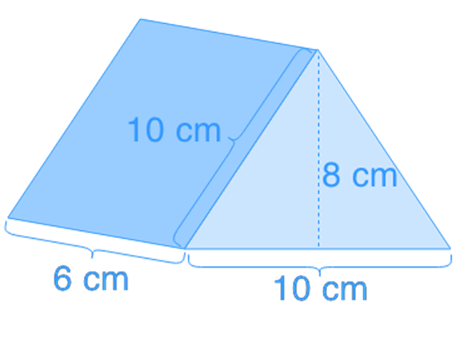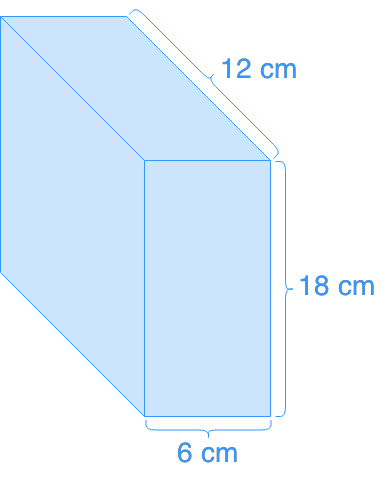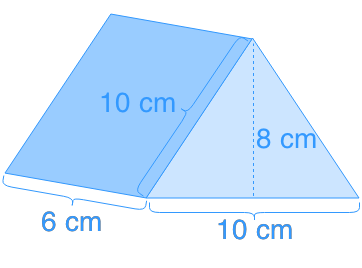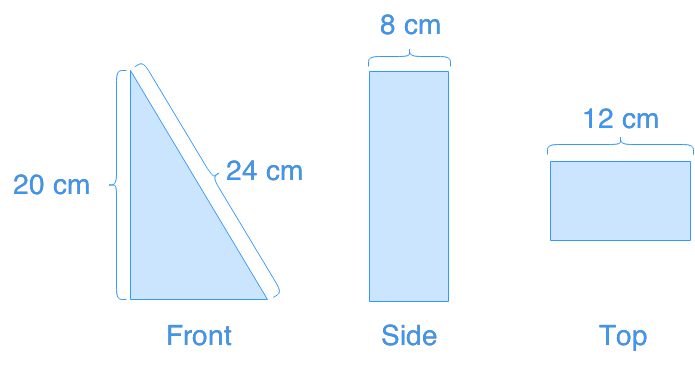# Surface area of prisms

## What is a triangular prism

To understand what a triangular prism is, let's start with the definition of prisms. Prisms are 3D shapes made of surfaces that are polygonal. They have polygonal bases on either sides which are connected to each other by rectangular or parallelogram surfaces. If it's connected with rectangular surfaces (its sides are made of rectangles), it's called a right prism. If it's connected by parallelograms, it's called an oblique prism.

The naming convention for prisms is to name the prism after the shape of its base. For example, we can have pentagonal prisms and square prisms. Today we're going to focus on triangular prisms, that is, a prism with a polygonal base that has 3 sides.

## How to find the surface area of a triangular prism

Area helps us find the amount of space contained on a 2D figure. So what is surface area?

3D objects have surface areas, which is the sum of the total area of the object's sides and faces. In order to find the surface area, the area of each of these sides and faces will have to be calculate and then added together. A good way to picture how this works is to use a net of a 3D figure. You can easily see how the surface area requires all the sides' area to be found and how it represents the total area surrounding the 3D figure.

Let's try to find the surface area of a triangular prism and take a look the prism below. We'll first divide up the steps to illustrate the concept of finding surface area, and then we'll give you the surface area of a triangular prism formula.

Question:

Find the surface area of the following triangular prism.3D triangular prism

Solution:

There are two triangles for its base (Front + Back). So calculate the triangle part of the surface area now:

$(\frac{10\; \times \;8}{2}) \times 2 = 80cm^2$

There are also three rectangles on the surface (Sides).

$(10 \; \times \; 6) \times 3 = 180cm^2$

What exactly happened in the above example? Firstly, you can identify all the sides of the triangular prism and their shapes. The shapes are important to figure out, since you know the formulas to finding the areas of basic shapes.

This triangular prism has two triangular bases and three rectangular sides. Firstly, to solve for the base triangles' areas, let's use the standard (base x height) / 2 formula for finding a triangle's area. This gives us $40cm^2$ per triangle. We then multiplied this by 2 since there are two bases. This gives us $80cm^2$.

Next, what's the area of a rectangle? It's just length times width, giving us $60cm^2$. Since there's three rectangles, multiply this number by 3, which gives us $180cm^2$.

Lastly, all you'll have do to find the total surface area of a triangular prism is to add together the areas of its sides, which gives you $260cm^2$.

If we wanted to condense the process to finding a triangular prism's surface area into a neat formula it would be the following:

$A=(\frac{1}{2})bh$

The formula for surface area of a triangular prism is actually a combination of the formulas for its triangular bases and rectangular sides. This online demonstration of an adjustable triangular prism is a good example to see the relationship between the object's height, lengths, and surface area.

### Surface area of prisms

In this section, we will learn how to calculate the surface area of rectangular and triangular prisms. The calculation is easier than what it may seem if you know the surface area formulas for regular polygons.

#### Lessons

• Introduction
a)
Intro to Surface Area
• What are rectangular prisms?
• What are triangular prisms?

• 1.
Find the surface area of the rectangular prism.• 2.
Find the surface area of the triangular prism.• 3.
Below are the views of a right triangular prism. Find the surface area.